This is an automatically generated documentation by LaTeX2HTML utility. In case of any issue, please, contact us at info@cfdsupport.com.

## System of Navier-Stokes equations in vector form

Starting from this section we will consider all the problems in two dimensions only. For better-arranged description, let us rewrite governing equations () in (two-dimensional) vector form: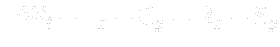(21.16)

where: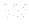denotes the vector of unknowns (conservative quantities),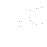are the vectors of inviscid (convective) fluxes,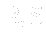are the vectors of viscous (diffuse) fluxes,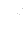is an additional matrix, that will be discussed.

Before using artificial compressibility method let us consider the vectors in the above equation have the following meaning: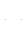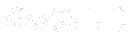(21.17)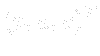(21.18)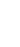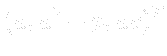(21.19)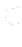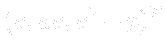(21.20)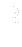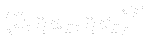(21.21)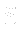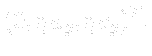(21.22)